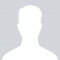Class Central is learner-supported. When you buy through links on our site, we may earn an affiliate commission.

# Regression Modeling in Practice

### Overview

##### Class Central Tips
This course focuses on one of the most important tools in your data analysis arsenal: regression analysis. Using either SAS or Python, you will begin with linear regression and then learn how to adapt when two variables do not present a clear linear relationship. You will examine multiple predictors of your outcome and be able to identify confounding variables, which can tell a more compelling story about your results. You will learn the assumptions underlying regression analysis, how to interpret regression coefficients, and how to use regression diagnostic plots and other tools to evaluate the quality of your regression model. Throughout the course, you will share with others the regression models you have developed and the stories they tell you.

### Syllabus

• Introduction to Regression
• This session starts where the Data Analysis Tools course left off. This first set of videos provides you with some conceptual background about the major types of data you may work with, which will increase your competence in choosing the statistical analysis that’s most appropriate given the structure of your data, and in understanding the limitations of your data set. We also introduce you to the concept of confounding variables, which are variables that may be the reason for the association between your explanatory and response variable. Finally, you will gain experience in describing your data by writing about your sample, the study data collection procedures, and your measures and data management steps.
• Basics of Linear Regression
• In this session, we discuss more about the importance of testing for confounding, and provide examples of situations in which a confounding variable can explain the association between an explanatory and response variable. In addition, now that you have statistically tested the association between an explanatory variable and your response variable, you will test and interpret this association using basic linear regression analysis for a quantitative response variable. You will also learn about how the linear regression model can be used to predict your observed response variable. Finally, we will also discuss the statistical assumptions underlying the linear regression model, and show you some best practices for coding your explanatory variables
Note that if your research question does not include one quantitative response variable, you can use one from your data set just to get some practice with the tool.
• Multiple Regression
• Multiple regression analysis is tool that allows you to expand on your research question, and conduct a more rigorous test of the association between your explanatory and response variable by adding additional quantitative and/or categorical explanatory variables to your linear regression model. In this session, you will apply and interpret a multiple regression analysis for a quantitative response variable, and will learn how to use confidence intervals to take into account error in estimating a population parameter. You will also learn how to account for nonlinear associations in a linear regression model. Finally, you will develop experience using regression diagnostic techniques to evaluate how well your multiple regression model predicts your observed response variable.
• Logistic Regression
• In this session, we will discuss some things that you should keep in mind as you continue to use data analysis in the future. We will also teach also you how to test a categorical explanatory variable with more than two categories in a multiple regression analysis. Finally, we introduce you to logistic regression analysis for a binary response variable with multiple explanatory variables. Logistic regression is simply another form of the linear regression model, so the basic idea is the same as a multiple regression analysis. But, unlike the multiple regression model, the logistic regression model is designed to test binary response variables. You will gain experience testing and interpreting a logistic regression model, including using odds ratios and confidence intervals to determine the magnitude of the association between your explanatory variables and response variable.
You can use the same explanatory variables that you used to test your multiple regression model with a quantitative outcome, but your response variable needs to be binary (categorical with 2 categories). If you have a quantitative response variable, you will have to bin it into 2 categories. Alternatively, you can choose a different binary response variable from your data set that you can use to test a logistic regression model. If you have a categorical response variable with more than two categories, you will need to collapse it into two categories.

Jen Rose

## Reviews

4.0 rating, based on 3 Class Central reviews

4.4 rating at Coursera based on 273 ratings

Start your review of Regression Modeling in Practice

• Naga Veera Mohan Kumar Ganavarapu completed this course.

•Alex Ivanov

Alex Ivanov completed this course.

• Colin Khein completed this course.

### Never Stop Learning.

Get personalized course recommendations, track subjects and courses with reminders, and more.